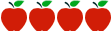### Grade 2: Understanding Measurement, Length, and Time

Rating:https://www.georgiastandards.org/Common-Core/Common%20Core%20Frameworks/CCGPS_Math_2_Unit3FrameworkSE.pdf

#### Common Core Standards

2.MD.1  Measure the length of an object by selecting and using appropriate tools such as rulers, yardsticks, meter sticks, and measuring tapes.

2.MD.2  Measure the length of an object twice, using length units of different lengths for the two measurements; describe how the two measurements relate to the size of the unit chosen.

2.MD.3  Estimate lengths using units of inches, feet, centimeters, and meters.

2.MD.4  Measure to determine how much longer one object is than another, expressing the length difference in terms of a standard length unit.

2.MD.5  Use addition and subtraction within 100 to solve word problems involving lengths that are given in the same units, e.g., by using drawings (such as drawings of rulers) and equations with a symbol for the unknown number to represent the problem.

2.MD.6  Represent whole numbers as lengths from 0 on a number line diagram with equally spaced points corresponding to the numbers 0, 1, 2, ..., and represent whole-number sums and differences within 100 on a number line diagram.

2.MD.7  Tell and write time from analog and digital clocks to the nearest five minutes, using a.m. and p.m.

2. MD.9 Generate measurement data by measuring lengths of several objects to the nearest whole unit, or by making repeated measurements of the same object. Show the measurements by making a line plot, where the horizontal scale is marked off in whole-number units.

2. MD.10 Draw a picture graph and a bar graph (with single- unit scale) to represent a data set with up to four categories. Solve simple put-together, take-apart, and compare problems1 using information presented in a bar graph.

#### Description of Unit

This unit “Understanding Measurement, Length, and Time” from the Georgia DOE contains curriculum-embedded CCSS aligned performance tasks and instructional supports. The mathematics of the unit moves second graders to measuring lengths with standard units - inches, feet, centimeters, and meters- using appropriate tools.

#### Cautions

Connecticut teachers should be aware that the unit is a high quality resource, but is missing the following components:

• Differentiation of Instruction: Explicit supports for English Language Learners or Special Needs students are not included; limited differentiation options are found in some lessons.
• Learning Extension: Not included for high interest/ ability students
• Integration of technology: Limited resources included

#### Rationale for Selection

• Content and instruction provided is of higher quality.
• The unit utilizes research-based instructional approaches.
• The scope and sequencing of unit is fairly easy to follow/implement.
• Broad differentiation and intervention strategies are provided within each activity.